# Robot Manipulation, Part 2: Dynamics and Control

In this blog post, Sebastian Castro will talk about robot manipulation with MATLAB and Simulink. The previous part discussed kinematics (if you have not read it, we recommend you do), while this part discusses dynamics.

– –

# Introduction

To motivate the importance of low-level robot manipulator control, I want to introduce a couple of engineering archetypes.

• Robot programmers usually start with a robot that has controllable joint or end effector positions. If you are a robot programmer, you are probably implementing motion planning algorithms and integrating the manipulator with other software components, such as perception and decision-making.
• Robot designers have a goal of enabling robot programmers. If you are a robot designer, you need to deliver a manipulator that can safely and reliably accept joint or end effector commands. You will likely apply some of the control design techniques discussed in this post and implement these controllers on embedded systems.

Of course, nothing is quite as rigidly separated in real life. Chances are that robot manufacturers will provide their own controllers, but may also decide to expose control parameters, options, or maybe even a direct interface to the actuator torques.

# From Kinematics to Dynamics

To recap the previous part, kinematics maps the joint positions of a robot manipulator to the positions and orientation of a coordinate frame of interest – usually, the end effector. Dynamics, on the other hand, maps the required joint forces and torques to their position, velocity, and acceleration.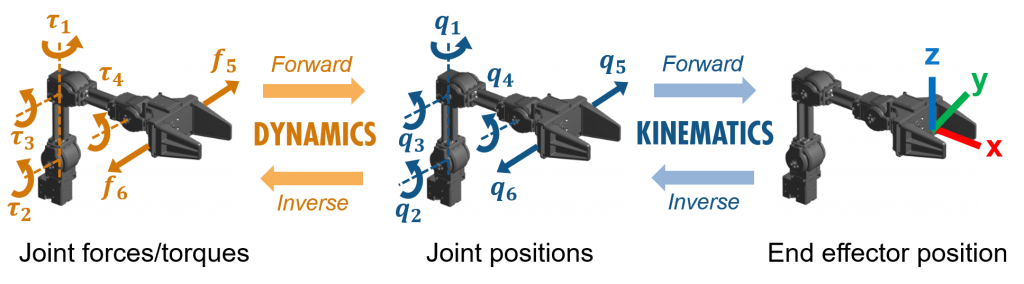To move from kinematics to dynamics, we need more information about the manipulator’s mechanics. Specifically, we need the following inertial properties:

• Mass: Newton’s second law relates mass to force and linear acceleration.
• Inertia: This is a 3×3 matrix, commonly called the inertia tensor, relating torque and angular acceleration. Since this matrix is skew-symmetric, it can be defined with 6 parameters:
• 3 diagonal elements, or moments of inertia, which relate torque about an axis with acceleration about that same axis.
• 3 off-diagonal elements, or products of inertia, which relate torque about an axis with acceleration about the other two axes.
• Center of mass: If the center of mass is not located at the body coordinate frame we defined, we need to apply the parallel-axis theorem to convert the rotations about the center of mass to rotations about our coordinate frame of interest.

Typically, you will import a robotics.RigidBodyTree from an existing manipulator description – for example, a URDF file. In this case, the inertial properties will be automatically placed in each robotics.RigidBody that comprises the tree.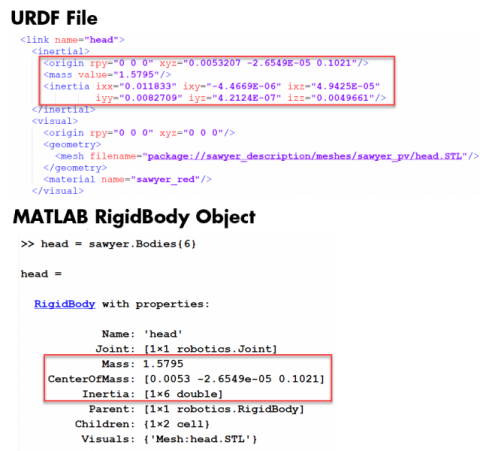# Controlling Joint Forces and Torques

A robot manipulator controller can contain the following components.

• Feedback: Uses desired and measured motion to compute joint inputs. This usually involves a control law that minimizes the error between the desired and measured motion.
• Feedforward: Uses desired motion only to compute joint inputs. This often – but not necessarily – involves a model of the manipulator mechanics to calculate an open-loop input.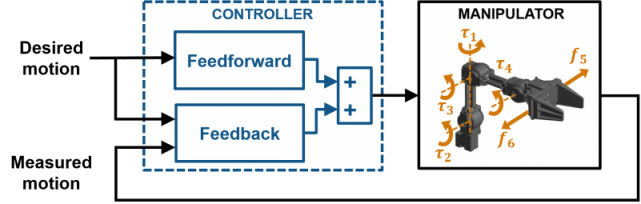In our video “Controlling Robot Manipulator Joints”, we explore two different examples of joint controllers, featuring the 4-DOF ROBOTIS OpenManipulator platform. You can also download the example files from the MATLAB Central File Exchange.

## Controller Example 1: Inverse Kinematics + Joint Space Controllers

First, inverse kinematics (IK) is used to convert the reference end effector position to a set of reference joint angles. The controller then operates exclusively in the configuration space – that is, on joint positions.

• The feedforward term uses inverse dynamics on our manipulator model. This calculates the required joint forces/torques such that the manipulator follows the desired motion, as well as compensates for gravity.
• The feedback term uses PID control. Each joint (4 revolute joints + gripper) has independent controllers that minimizes the error between desired and measured motion.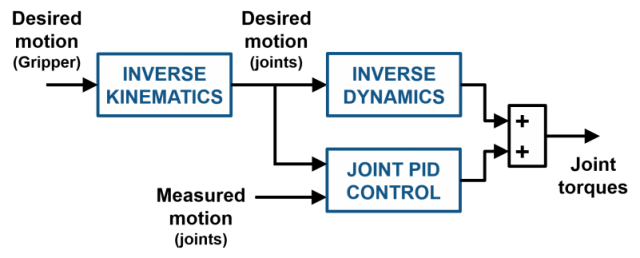For smooth motion, we typically want a closed-form trajectory such as a curve equation. This is because inverse dynamics requires positions, velocities, and accelerations to calculate required joint forces/torques. So, having a differentiable reference trajectory makes this much easier.

Theoretically, inverse dynamics should be enough to control a robot arm. However, there are factors such as joint mechanics (stiffness, damping, friction, etc.), unmeasurable disturbances, sensor/actuator noise, or even numerical error, that can easily impact the robustness of a fully open-loop controller. Therefore, an additional feedback compensator is always recommended.

While the feedforward and feedback control portions are relatively easy to implement and computationally inexpensive, this controller structure relies on solving IK. As we discussed in the previous part, the Robotics System Toolbox implementation uses a numerical solution and therefore can require significant computation. You can address this by providing a good initial guess (usually the previous measurement), limiting the maximum number of iterations, or switching to an analytical IK solution.

## Controller Example 2: Task Space Controller

This second controller performs the control in the task space – that is, on the end effector positions and orientations. In addition, it avoids the need for inverse kinematics by using the geometric Jacobian.

The geometric Jacobian is a function of the robot configuration q (joint angles/positions), which is why it is often denoted as J(q). The Jacobian is a mapping from the joint velocities to the world velocities of a coordinate frame of interest. However, with a bit of math you can find that it also maps the joint forces/torques to world forces/torques. I found this blog post to be a helpful reference.

• The feedforward term in this controller only does one thing: compensates for gravity.
• The feedback term performs PID control on the XYZ positions of the end effector (we ignore orientation here, but you really shouldn’t!) to calculate desired forces at the end effector coordinate frame. Then, the Jacobian converts the control outputs to joint torques and forces.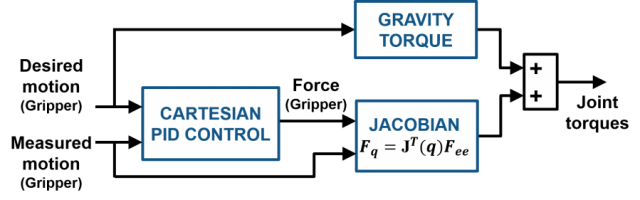Below is a screenshot of the Simulink model for this example controller. Unlike the schematic above, the model contains other realistic artifacts such as filters, rate limiters, saturation, and a decoupled gripper controller with basic logic. You can download this model from the MATLAB Central File Exchange.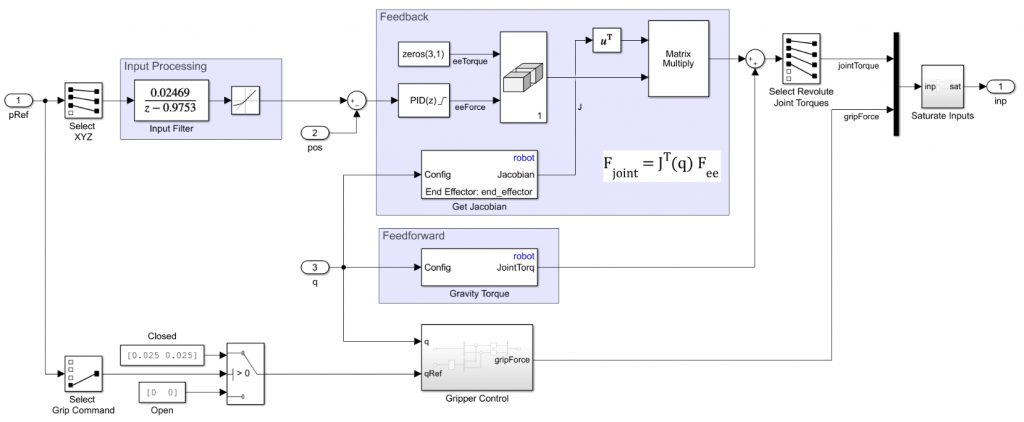# Survey of Control Design Techniques

Once you have a model of your manipulator, there are many tools in MATLAB and Simulink that can help you design joint controllers. These include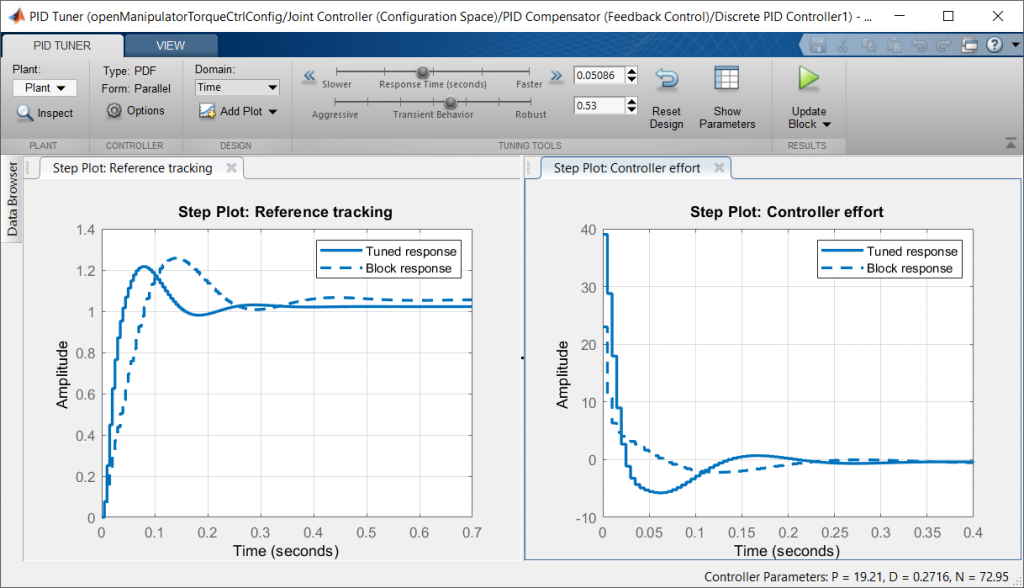PID Tuner output on the “shoulder” joint of the ROBOTIS OpenManipulator model

Traditional control design relies on linearization, or finding a linear approximate of a nonlinear model about a specific operating point – for example, the “home”, or equilibrium, position of the manipulator. A controller designed about an approximate linear region can become less effective, and potentially unstable, as the robot state deviates from that region.

Nonlinear control techniques can address this issue by considering the measured state of the system (in our case, joint or end effector positions). Feedforward techniques like inverse dynamics, or calculating the geometric Jacobian on the fly, can ensure that the controller accounts for nonlinearities in the model. Another popular technique is gain scheduling, which can be used for both traditional controllers and MPC controllers.

Another alternative is to employ model-free techniques such as:

• Optimization: You can optimize control parameters using simulation, which is enabled by Simulink Design Optimization. While optimization makes no guarantees on stability, it lets you automatically tune a breadth of parameters such as gains, control effort/rate limits, thresholds, etc. which could lead to good results – especially on highly nonlinear systems.
• Machine learning: Reinforcement learning, or automatically learning by trial and error, is a common technique being employed for robot manipulation. For example, this paper and video shows deep reinforcement learning – in other words, learning parameters of a deep neural network using reinforcement learning techniques.

# Conclusion

Now you’ve seen an overview of kinematics and dynamics for robot manipulator design. I hope this was a useful introduction to the language in this domain, some common techniques used in practice, and areas where MATLAB and Simulink can help you design and control robots.

We hope that Simulink can help you during your design phase as you explore different architectures, integrate supervisory logic, perform tradeoff studies, and more. Also, recall that Simulink lets you automatically generate standalone C/C++ code from your control algorithms, so they can be deployed to hardware or middleware such as ROS.

If you want to see more material on robot manipulation, or other topics in robotics, feel free to leave us a comment or email us at roboticsarena@mathworks.com. I hope you enjoyed reading!

– Sebastian

|